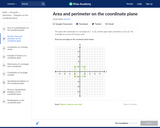Updating search results...

# 440 Results

View
Selected filters:
• geometry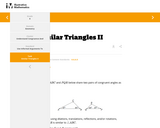Unrestricted Use
CC BY
Rating
0.0 stars

This is a task from the Illustrative Mathematics website that is one part of a complete illustration of the standard to which it is aligned. Each task has at least one solution and some commentary that addresses important aspects of the task and its potential use.

Subject:
Mathematics
Material Type:
Activity/Lab
Provider:
Illustrative Mathematics
Provider Set:
Illustrative Mathematics
Author:
Illustrative Mathematics
05/03/2019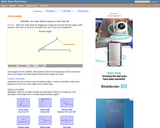Rating
0.0 stars

An interactive applet and associated web page that demonstrate acute angles (those less than 90 deg). The applet presents an angle (initially acute) that the user can adjust by dragging the end points of the line segments forming the angle. As it changes it shows the angle measure and a message that indicate which type of angle it is. There a software 'detents' that make it easy capture exact angles such as 90 degrees and 180 degrees The message and angle measures can be turned off to facilitate classroom discussion. The text on the page has links to other pages defining each angle type in depth. Applet can be enlarged to full screen size for use with a classroom projector. This resource is a component of the Math Open Reference Interactive Geometry textbook project at http://www.mathopenref.com.

Subject:
Geometry
Mathematics
Material Type:
Simulation
Provider:
Math Open Reference
Author:
John Page
05/07/2019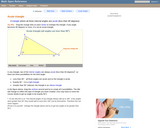Rating
0.0 stars

An interactive applet and associated web page that demonstrate the three types of triangle: acute, obtuse and right. The applet shows a triangle that is initially acute (all angles less then 90 degrees) which the user can reshape by dragging any vertex. There is a message changes in real time while the triangle is being dragged that tells if the triangle is an acute, right or obtuse triangle and gives the reason why. By experimenting with the triangle student can develop an intuitive sense of the difference between these three classes of triangle. Applet can be enlarged to full screen size for use with a classroom projector. This resource is a component of the Math Open Reference Interactive Geometry textbook project at http://www.mathopenref.com.

Subject:
Geometry
Mathematics
Material Type:
Simulation
Provider:
Math Open Reference
Author:
John Page
05/07/2019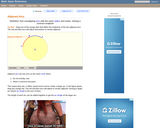Rating
0.0 stars

An interactive applet and associated web page that demonstrate the the concept of adjacent arcs. The user can 3 drag points around a circle that define the endpoints of two adjacent arcs. Web page has the properties of adjacent arcs. Applet can be enlarged to full screen size for use with a classroom projector. This resource is a component of the Math Open Reference Interactive Geometry textbook project at http://www.mathopenref.com.

Subject:
Geometry
Mathematics
Material Type:
Simulation
Provider:
Math Open Reference
Author:
John Page
05/07/2019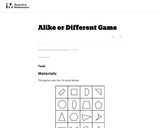Unrestricted Use
CC BY
Rating
0.0 stars

In this game activity students practice comparing shapes and naming something that is alike or different about them.

Subject:
Mathematics
Material Type:
Activity/Lab
Provider:
Illustrative Mathematics
Provider Set:
Illustrative Mathematics
Author:
Illustrative Mathematics
05/01/2012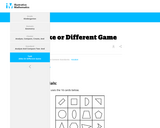Rating
0.0 stars

For this game, students work in pairs drawing two cards and naming something that is ALIKE or DIFFERENT between the two cards. The pairs draw two more cards and repeat the process until there are no more cards. The game can be played whole group or as a competition.

Subject:
Mathematics
Material Type:
Activity/Lab
Provider:
Illustrative Mathematics
Author:
Illustrative Mathematics
02/26/2019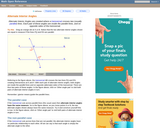Rating
0.0 stars

An interactive applet and associated web page that demonstrate the alternate interior angles that are formed where a transversal crosses two lines. The applets shows the two possible pairs of angles alternating when in animation mode. By dragging the three lines, it can be seen that the angles are congruent only when the lines are parallel. When not in animated mode, there is a button that alternates the two pairs of angles. The text on the page discusses the properties of the angle pairs both in the parallel and non-parallel cases. Applet can be enlarged to full screen size for use with a classroom projector. This resource is a component of the Math Open Reference Interactive Geometry textbook project at http://www.mathopenref.com.

Subject:
Geometry
Mathematics
Material Type:
Simulation
Provider:
Math Open Reference
Author:
John Page
05/07/2019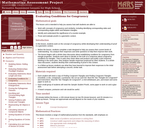Only Sharing Permitted
CC BY-NC-ND
Rating
0.0 stars

This lesson unit is intended to help teachers assess how well students are able to: work with concepts of congruency and similarity, including identifying corresponding sides and corresponding angles within and between triangles; Identify and understand the significance of a counter-example; Prove, and evaluate proofs in a geometric context.

Subject:
Geometry
Mathematics
Material Type:
Assessment
Lesson Plan
Provider:
Shell Center for Mathematical Education
Provider Set:
Mathematics Assessment Project (MAP)
06/24/2019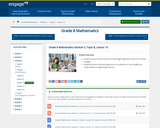Conditional Remix & Share Permitted
CC BY-NC-SA
Rating
0.0 stars

In this lesson, students know an informal proof of the Angle-Angle (AA) criterion for similar triangles. Students present informal arguments as to whether or not triangles are similar based on Angle-Angle criterion.

Subject:
Mathematics
Material Type:
Lesson Plan
Provider:
EngageNY
02/16/2017Rating
0.0 stars

Students work with a partner and sit opposite one another with a divider between them. Each student takes turns drawing and labeling angles in each space on their grids and give instructions to their partner on how to draw the angles to match the ones on his/her grid. Students use positional language to describe where to place the angles.

Subject:
Mathematics
Material Type:
Activity/Lab
Provider:
K-5 Math Teaching Resources
Author:
K-5 Math Teaching Resources
02/26/2019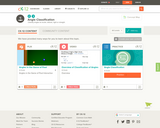Conditional Remix & Share Permitted
CC BY-NC-SA
Rating
0.0 stars

This short video and interactive assessment activity is designed to give fourth graders an overview of classification of angles.

Subject:
Mathematics
Material Type:
Assessment
Interactive
Lecture
Provider:
CK-12 Foundation
Provider Set:
CK-12 Elementary Math
06/06/2019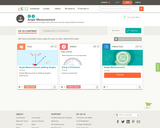Conditional Remix & Share Permitted
CC BY-NC-SA
Rating
0.0 stars

This short video and interactive assessment activity is designed to give fourth graders an overview of measurement of angles.

Subject:
Mathematics
Material Type:
Assessment
Interactive
Lecture
Provider:
CK-12 Foundation
Provider Set:
CK-12 Elementary Math
06/06/2019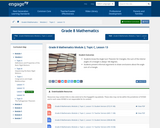Conditional Remix & Share Permitted
CC BY-NC-SA
Rating
0.0 stars

In this lesson, students know the Angle Sum Theorem for triangles; the sum of the interior angles of a triangle is always 180 degrees. Students present informal arguments to draw conclusions about the angle sum of a triangle.

Subject:
Mathematics
Material Type:
Lesson Plan
Provider:
EngageNY
02/16/2017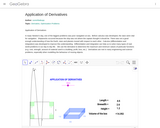Conditional Remix & Share Permitted
CC BY-SA
Rating
0.0 stars

We use the derivative to determine the maximum and minimum values of particular functions (e.g. cost, strength, amount of material used in a building, profit, loss, etc.).Differentiation is also used in analysis of finance and economics.

Subject:
Geometry
Mathematics
Material Type:
Simulation
Provider:
GeoGebra
Provider Set:
GeoGebraTube
03/12/2012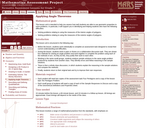Only Sharing Permitted
CC BY-NC-ND
Rating
0.0 stars

This lesson unit is intended to help you assess how well students are able to use geometric properties to solve problems. In particular, it will support you in identifying and helping students who have the following difficulties: Solving problems relating to using the measures of the interior angles of polygons; and solving problems relating to using the measures of the exterior angles of polygons.

Subject:
Geometry
Mathematics
Material Type:
Assessment
Lesson Plan
Provider:
Shell Center for Mathematical Education
Provider Set:
Mathematics Assessment Project (MAP)
06/24/2019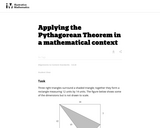Unrestricted Use
CC BY
Rating
0.0 stars

This task requires students to apply the Pythagorean Theorem.

Subject:
Mathematics
Material Type:
Activity/Lab
Provider:
Illustrative Mathematics
Provider Set:
Illustrative Mathematics
Author:
Illustrative Mathematics
05/01/2012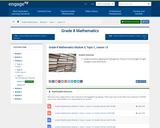Conditional Remix & Share Permitted
CC BY-NC-SA
Rating
0.0 stars

In this lesson, students practice applying the Pythagorean Theorem to find lengths of right triangles in two dimensions.

Subject:
Mathematics
Material Type:
Lesson Plan
Provider:
EngageNY
02/16/2017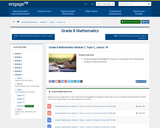Conditional Remix & Share Permitted
CC BY-NC-SA
Rating
0.0 stars

For this lesson, students apply the Pythagorean Theorem to real world and mathematical problems in two dimensions.

Subject:
Mathematics
Material Type:
Lesson Plan
Provider:
EngageNY
02/24/2017Conditional Remix & Share Permitted
CC BY-NC-SA
Rating
0.0 stars

This short video and interactive assessment activity is designed to give fourth graders an overview of composite figures composed of squares and rectangles.

Subject:
Mathematics
Material Type:
Assessment
Interactive
Lecture
Provider:
CK-12 Foundation
Provider Set:
CK-12 Elementary Math
06/06/2019